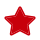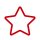# 入门示例

#### 算子分析

1. 明确算子实现文件名称、算子实现函数名称以及算子的OpType。

命名规则如下：

• 算子类型需要采用大驼峰的命名方式，即采用大写字符区分不同的语义。
• 算子文件名称和算子函数名称，可选用以下任意一种命名规则：
• 用户自定义，此时需要在算子信息定义中配置opFile.valueopInterface.value
• 不配置算子信息定义中的opFile.valueopInterface.value，FE会将OpType按照如下方式进行转换后进行算子文件名和算子函数名的匹配。
转换规则如下：
• 首字符的大写字符转换为小写字符。

例如：Abc -> abc

• 小写字符后的大写字符转换为下划线+小写字符。

例如：AbcDef -> abc_def

• 紧跟数字以及大写字符后的大写字符，作为同一语义字符串，查找此字符串后的第一个小写字符，并将此小写字符的前一个大写字符转换为下划线+小写字符，其余大写字符转换为小写字符。若此字符串后不存在小写字符，则直接将此字符串中的大写字符转换为小写字符。

例如：ABCDef -> abc_def；Abc2DEf -> abc2d_ef；Abc2DEF -> abc2def；ABC2dEF -> abc2d_ef。

2. 明确算子的功能以及数学表达式。

该算子用于实现两个tensor的element-wise相加。

数学表达式为：C = A + B。

3. 明确使用哪些TIK计算接口。
• 定义数据。需要使用Tensor接口。
• 将输入数据从Global Memory搬入Unified Buffer。需要使用data_move接口。
• 将得到的结果从Unified Buffer搬出到Global Memory。需要使用data_move接口。

#### 算子实现1. Python模块导入。
2. 定义目标机，并构建TIK DSL容器。
3. 通过数据定义接口在AI Core的外部存储（例如Global Memory）和内部存储（例如Unified Buffer）中定义输入数据、输出数据。
4. 通过数据搬运接口将数据从AI Core的外部存储搬运到内部存储中。
5. 通过标量计算、矢量计算和矩阵计算等接口进行数据计算。
6. 通过数据搬运接口将数据从AI Core的内部存储搬运到外部存储中。

由于Unified BufferAI Core内部存储空间有限，当数据量大时无法完整放入输入数据和输出结果，需要对输入数据分片搬入、计算、再搬出。

7. 进行算子编译，最终生成算子目标文件.o与算子描述文件.json。

 ``` 1 2 3 4 5 6 7 8 9 10 11 12 13 14 15 16 17 18 19 20 21 22``` ```from te import tik tik_instance = tik.Tik() data_A = tik_instance.Tensor("float16", (128,), name="data_A", scope=tik.scope_gm) data_B = tik_instance.Tensor("float16", (128,), name="data_B", scope=tik.scope_gm) data_C = tik_instance.Tensor("float16", (128,), name="data_C", scope=tik.scope_gm) data_A_ub = tik_instance.Tensor("float16", (128,), name="data_A_ub", scope=tik.scope_ubuf) data_B_ub = tik_instance.Tensor("float16", (128,), name="data_B_ub", scope=tik.scope_ubuf) data_C_ub = tik_instance.Tensor("float16", (128,), name="data_C_ub", scope=tik.scope_ubuf) tik_instance.data_move(data_A_ub, data_A, 0, 1, 128 //16, 0, 0) tik_instance.data_move(data_B_ub, data_B, 0, 1, 128 //16, 0, 0) tik_instance.vec_add(128, data_C_ub, data_A_ub, data_B_ub, 1, 8, 8, 8) tik_instance.data_move(data_C, data_C_ub, 0, 1, 128 //16, 0, 0) tik_instance.BuildCCE(kernel_name="simple_add",inputs=[data_A,data_B],outputs=[data_C]) return tik_instance ```

1. 导入Python模块。

 `1` ```from te import tik ```

“te.tik”：提供了所有TIK相关的python函数，具体请参考ATC安装目录下的“python/site-packages/te.egg/te/tik”

2. 构造TIK DSL容器。

通过TIK类构造函数构造TIK DSL容器。
 `1` ```tik_instance = tik.Tik() ```

3. 定义数据。

通过TensorGlobal Memory中定义输入数据data_A和data_B，输出数据data_C。他们的Size分别为128个float16类型的数据。

通过TensorUnified Buffer中定义数据data_A_ub、data_B_ub、data_C_ub。他们的Size分别为128个float16类型的数据。

• 【接口定义】Tensor(dtype, shape, scope, name)
• 【参数分析】
• dtype：指定Tensor对象的数据类型。
• shape：指定Tensor对象的形状。
• scope：指定Tensor对象的所在buffer空间。scope_gm表示Global Memory中的数据；scope_ubuf表示Unified Buffer中的数据。
• name：指定Tensor名字，不同Tenso名字需要保持唯一。
• 【示例】
 ```1 2 3 4``` ```#在Global Memory中定义输入数据data_A和data_B，输出数据data_C。他们的Size分别为128个float16类型的数据。 data_A = tik_instance.Tensor("float16", (128,), name="data_A", scope=tik.scope_gm) data_B = tik_instance.Tensor("float16", (128,), name="data_B", scope=tik.scope_gm) data_C = tik_instance.Tensor("float16", (128,), name="data_C", scope=tik.scope_gm) ```
 ```1 2 3 4``` ```#在Unified Buffer中定义数据data_A_ub、data_B_ub、data_C_ub。他们的Size分别为128个float16类型的数据。 data_A_ub = tik_instance.Tensor("float16", (128,), name="data_A_ub", scope=tik.scope_ubuf) data_B_ub = tik_instance.Tensor("float16", (128,), name="data_B_ub", scope=tik.scope_ubuf) data_C_ub = tik_instance.Tensor("float16", (128,), name="data_C_ub", scope=tik.scope_ubuf) ```

4. Global Memory中的数据搬运到Unified Buffer中。

通过data_move实现数据搬运，即将data_A中的数据搬运到data_A_ub，data_B中的数据搬运到data_B_ub。
• 【接口定义】data_move (dst, src, sid, nburst, burst, src_stride, dst_stride, *args, **argv)
• 【参数分析】
• src, dst：源地址与目标地址。
• sid：sim ID，固定为0。
• burst, nburst：burst为每次搬运的数据大小（单位为32Byte），nburst为搬运次数。我们需要搬运的数据为128个float16类型的数据，占128*2Byte，小于Unified Buffer的大小（256KB），因此我们搬一次就可以把输入数据全部搬到Unified Buffer，搬运的次数nburst为1；由于burst单位为32Byte，每次搬运的数据大小burst为128*2/32Byte。
• src_stride, dst_stride：源/目的地址的stride，当需要带间隔跳跃式搬运时，需要设置这两个参数，示例中为连续搬运，因此两个参数都设置为0。
• 【示例】
 ```1 2``` ```tik_instance.data_move(data_A_ub, data_A, 0, 1, 128 //16, 0, 0) tik_instance.data_move(data_B_ub, data_B, 0, 1, 128 //16, 0, 0) ```

在实现计算之前，我们先来了解下TIK指令的基本操作单位。

• 【参数分析】
• src0，src1，dst：源操作数0，源操作数1和目的操作数，分别为data_A_ub、data_B_ub、data_C_ub。
• repeat_times：迭代次数。通过上面对TIK指令的基本了解，我们可以算出，对于128个float16的数据，通过1次repeat可以完成计算，因此repeat_times为1。
• dst_rep_stride, src0_rep_stride, src1_rep_stride：相邻迭代间，目的操作数/源操作数0/源操作数1头头间地址间隔，单位为32Byte，此处配置为8，表示一个迭代连续处理8*32Byte数据。
• 【示例】
 `1` ```tik_instance.vec_add(128, data_C_ub, data_A_ub, data_B_ub, 1, 8, 8, 8) ```

6. 将data_C_ub中的计算结果搬运到data_C中。同样使用data_move实现，和4的分析过程类似，不再赘述。

 `1` ```tik_instance.data_move(data_C, data_C_ub, 0, 1, 128 //16, 0, 0) ```

7. 将TIK DSL容器中的语句，编译成昇腾AI处理器可执行的代码。

通过BuildCCE将上述定义的TIK DSL容器编译生成昇腾AI处理器的可执行二进制文件。

• 【接口定义】BuildCCE(kernel_name, inputs, outputs, output_files_path=None, enable_l2=False)
• 【参数分析】
• kernel_name：指明编译产生的二进制代码中的AI Core核函数名称。
• inputs：存放程序的输入Tensor，必须是Global Memory的存储类型。
• outputs：存放程序的输出Tensor，必须是Global Memory的存储类型。
• output_files_path：指明编译产生文件的存储位置，默认为”./kernel_meta”。
• enable_l2：该参数暂不生效。
• 【示例】
 `1` ```tik_instance.BuildCCE(kernel_name="simple_add",inputs=[data_A,data_B],outputs=[data_C]) ```

8. 返回tik实例。

 `1` ```return tik_instance ```

# 相关产品